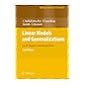Normal view

# Linear models and generalizations : least squares and alternatives / C. Radhakrishna Rao ... [et al.]

Material type:TextLanguage: English Publisher: New York : Springer, 2008Edition: 3rd extended edDescription: xix, 570 p. : ill. ; 25 cmISBN: 9783540742265 ; 9780387988481Subject(s): Mathematical satistics | Linear models (Statistics)DDC classification: 519.5 Online resources: Ebook Fulltext
Contents:
Table of contents 1. Introduction -- 2. The Simple Linear Regression Model -- 3. The Multiple Linear Regression Model -- 4. The Generalized Linear Regression Model -- 5. Exact and Stochastic Linear Restrictions -- 6. Prediction Problems in the Generalized Regression Model -- 7. Sensitivity Analysis -- 8. Analysis of Incomplete Data Sets -- 9. Robust Regression -- 10. Models for Categorical Response Variables -- Fitting Smooth Functions -- Appendix A: Matrix Algebra
Summary: "This book provides an up-to-date account of the theory and applications of linear models. The authors present a unified theory of inference from linear models and its generalizations with minimal assumptions, not only through least squares theory, but also using alternative methods of estimation and testing based on convex loss functions and general estimating equations. It can be used as a text for courses in Read more...
Tags from this library: No tags from this library for this title.Average rating: 0.0 (0 votes)
Item type Current location Collection Call number Copy number Status Date due Barcode Item holdsE-Book
E-book
Non-fiction 519.5 LIN 2008 (Browse shelf) Not for loanText
Reserve Section
Non-fiction 519.5 LIN 2008 (Browse shelf) C-1 Not For Loan 27312Text
Circulation Section
Non-fiction 519.5 LIN 2008 (Browse shelf) C-2 Available 28167
Total holds: 0
##### Browsing EWU Library shelves, Shelving location: Circulation Section Close shelf browser519.5 LES 1997 Statistics for management / 519.5 LES 1997 Statistics for management / 519.5 LES 1997 Statistics for management / 519.5 LIN 2008 Linear models and generalizations : 519.5 LIS Statistical techniques in business & economics / 519.5 LIS Statistical techniques in business & economics / 519.5 LIS 2001 Statistical techniques in business & economics /

Print version:
Linear models and generalizations.
Berlin ; New York : Springer, ©2008
(OCoLC)173807301.

Includes Bibliographical References and Index.

2. The Simple Linear Regression Model --
3. The Multiple Linear Regression Model --
4. The Generalized Linear Regression Model --
5. Exact and Stochastic Linear Restrictions --
6. Prediction Problems in the Generalized Regression Model --
7. Sensitivity Analysis --
8. Analysis of Incomplete Data Sets --
9. Robust Regression --
10. Models for Categorical Response Variables --
Fitting Smooth Functions --
Appendix A: Matrix Algebra

"This book provides an up-to-date account of the theory and applications of linear models. The authors present a unified theory of inference from linear models and its generalizations with minimal assumptions, not only through least squares theory, but also using alternative methods of estimation and testing based on convex loss functions and general estimating equations. It can be used as a text for courses in Read more...

Applied Statistics

There are no comments for this item.